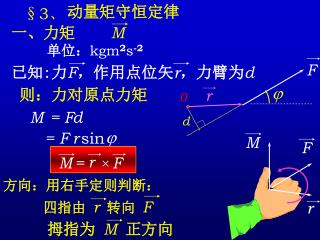DownloadDownload PresentationÂ§ 3 ã€

# Â§ 3 ã€

Télécharger la présentation## Â§ 3 ã€

- - - - - - - - - - - - - - - - - - - - - - - - - - - E N D - - - - - - - - - - - - - - - - - - - - - - - - - - -
##### Presentation Transcript

1. §3、 动量矩守恒定律 一、力矩 M 已知:力F，作用点位矢r，力臂为d M = F d = F r sin j M F F r M = F × j r 0 r 四指由 r转向 F d 拇指为 M正方向 单位：kgm2s-2 则：力对原点力矩 方向：用右手定则判断：

2. 二、动量矩 L 已知: 质点质量为m,位矢为r，速度为v mv P L = = m P v d j r 0 m d L P r L = P = r × mv × r 比较 r M = F × 单位：kgm2s-1 则：质点的动量 则：对原点的动量矩 = mv r sinj 动量矩 力矩 方向：用右手定则判断。

3. 三、 动量矩守恒定律 r ( r×P ) d d L L mv d d d = = mv + r × × dt dt dt dt dt = v × mv + r ×F = M M 0 = c L = 则： 即： = 0 若： 若系统的给定点的所受外力矩为零， 系统对该定点的动量矩守恒

4. L d dt d P M = = F d t 即：系统的合外力为零，总动量守恒。 即：若系统的给定点所受外力矩为零， 则系统对该定点的动量矩守恒

5. 质量为m的质点以速度沿一直线运动， 则它对直线上任一点的角动量为何值？ m m A r r r L L = = P = r × mv P = r × mv × × v A d = mv r sinj L v j [例1] = 0 [例2]质量为m的质点以速度沿一直线运动， 则它对与直线垂直距离为d的一点的角动量 大小是多少？ = mvd

6. 已知地球的质量为m，太阳的质量为M， 地心与日心的距离为 R，引力常数为 G， 求：地球绕太阳作圆周运动的轨道角动量。 解： r L = P = r × mv × F = G = m v2 vR = GMR Mm R R2 = m GMR [例3] L = R mv

7. F 30 103 由图得： F = 30- ( t-3 )2 t 0 3 6 t = 3 - 3 = 1.27 s 质量的木箱在水平拉力F的作用下，由静止开始运动，若F随时间的变化关系如图中抛物线所表示那样，若木箱与地面之间的静摩擦系数，滑动摩擦系数，如重力加速度以计算，求： (1)写出力F随时间的变化函数；(2)木箱何时开始运动？ (3)6s时木箱的运动速度。 [2-36] 解：设： F = a + b( t+c )2 (2) F = m1mg = 20 N 解得: 木箱开时开始运动

8. 6 ò I =DP = F合dt 1.27 103 109 = 20t- ( t-3 )3 = 20- ( t-3 )2 = 30 +50 3 /3 F合= F – m2mg (3) = 59 Ns v = I/m = 5.9 m/s